# Api 接口优化的几个技巧

0收藏## 哪些问题会引起接口性能问题？

• 数据库慢查询
• 深度分页问题
• 未加索引
• 索引失效
• join过多
• 子查询过多
• in中的值太多
• 单纯的数据量过大
• 业务逻辑复杂
• 循环调用
• 顺序调用
• 线程池设计不合理
• 锁设计不合理
• 机器问题（fullGC，机器重启，线程打满）

## 1、慢查询（基于mysql）

### 1.1 深度分页

``select name,code from student limit 100,20``

``select name,code from student limit 1000000,20``

``select name,code from student where id>1000000  limit 20``

### 1.2 未加索引

``show create table xxxx（表名）``

### 1.3 索引失效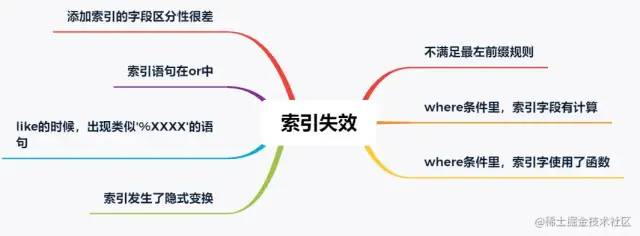• 某个字段只可能有3个值，那这个字段的索引区分度就很低。
• 再比如，某个字段大量为空，只有少量有值；
• 再比如，某个字段值非常集中，90%都是1，剩下10%可能是2,3,4....

``select name,code from student force index(XXXXXX) where name = '天才' ``

### 1.5 in的元素过多

``select id from student where id in (1,2,3 ...... 1000) limit 200``

``````if (ids.size() > 200) {
throw new Exception("单次查询数据量不能超过200");
}``````

## 2、业务逻辑复杂

### 2.1 循环调用

``````List<Model> list = new ArrayList<>();
for(int i = 0 ; i < 12 ; i ++) {
Model model = calOneMonthData(i); // 计算某个月的数据，逻辑比较复杂，难以批量计算，效率也无法很高
}``````

``````// 建立一个线程池，注意要放在外面，不要每次执行代码就建立一个，具体线程池的使用就不展开了

// 开始多线程调用
List<Future<Model>> futures = new ArrayList<>();
for(int i = 0 ; i < 12 ; i ++) {
Future<Model> future = commonThreadPool.submit(() -> calOneMonthData(i););
}

// 获取结果
List<Model> list = new ArrayList<>();
try {
for (int i = 0 ; i < futures.size() ; i ++) {
}
} catch (Exception e) {
LOGGER.error("出现错误：", e);
}``````

### 2.2 顺序调用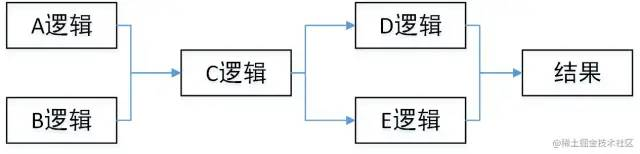``````A a = doA();
B b = doB();

C c = doC(a, b);

D d = doD(c);
E e = doE(c);

return doResult(d, e);``````

``````CompletableFuture<A> futureA = CompletableFuture.supplyAsync(() -> doA());
CompletableFuture<B> futureB = CompletableFuture.supplyAsync(() -> doB());
CompletableFuture.allOf(futureA,futureB) // 等a b 两个任务都执行完成

C c = doC(futureA.join(), futureB.join());

CompletableFuture<D> futureD = CompletableFuture.supplyAsync(() -> doD(c));
CompletableFuture<E> futureE = CompletableFuture.supplyAsync(() -> doE(c));
CompletableFuture.allOf(futureD,futureE) // 等d e两个任务都执行完成

return doResult(futureD.join(),futureE.join());``````

## 3、线程池设计不合理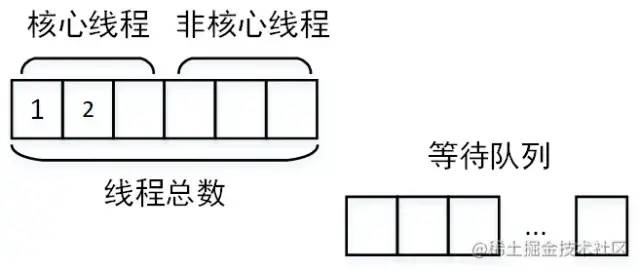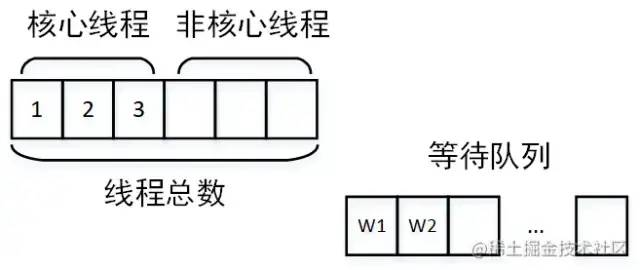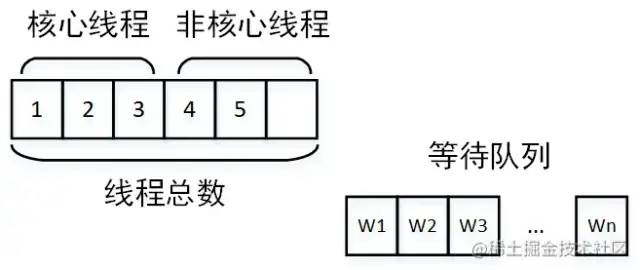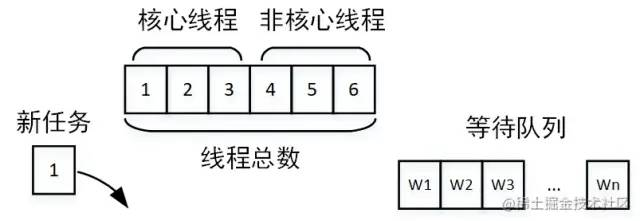• 核心线程设置过小：核心线程设置过小则没有达到并行的效果
• 线程池公用，别的业务的任务执行时间太长，占用了核心线程，另一个业务的任务到达就直接进入了等待队列
• 任务太多，以至于占满了线程池，大量任务在队列中等待

## 4、锁设计不合理

``````public synchronized void doSome(){
File f = calData();
sendSuccessMessage();
}``````

``````public void doSome(){
File f = null;
synchronized(this) {
f = calData();
}
sendSuccessMessage();
}``````

## 6、万金油解决方式

### 6.1 缓存

• 简单的​`​map​`
• `​guava​`​等本地缓存工具包
• 缓存中间件：​`​redis​`​​、​`​tair​`​​或​`​memcached​`

### 6.2 回调 or 反查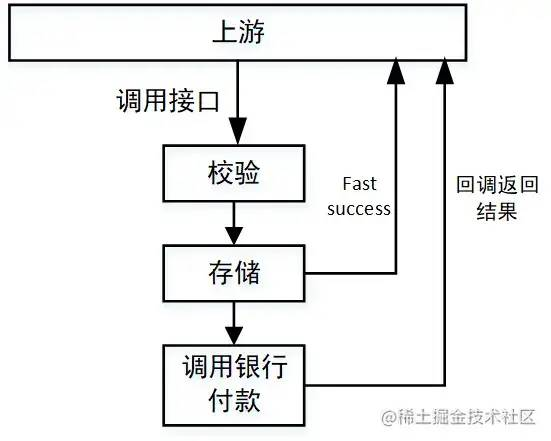​​来源：juejin.cn/post/7043423820543164453